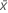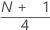# Methods and formulas for Display Descriptive Statistics

Select the method or formula of your choice.

## Mean

A commonly used measure of the center of a batch of numbers. The mean is also called the average. It is the sum of all observations divided by the number of (nonmissing) observations.

### Notation

TermDescription
xiith observation
Nnumber of nonmissing observations

## Standard error of the mean (SE Mean)

The standard error of the mean is calculated as the standard deviation divided by the square root of the sample size.

### Notation

TermDescription
s standard deviation of the sample
N number of nonmissing observations

## Standard deviation (StDev)

The sample standard deviation provides a measure of the spread of your data. It is equal to the square root of the sample variance.

### Formula

If the column contains x 1, x 2,..., x N, with mean, then the standard deviation of the sample is:

### Notation

TermDescription
x i i th observationmean of the observations
N number of nonmissing observations

## Variance

The variance measures how spread out the data are about their mean. The variance is equal to the standard deviation squared.

### Notation

TermDescription
xiith observationmean of the observations
Nnumber of nonmissing observations

## Coefficient of variation (CoefVar)

The coefficient of variation is a measure of relative variability calculated as a percentage.

### Formula

Minitab calculates it as:

### Notation

TermDescription
s standard deviation of the samplemean of the observations

## 1st quartile (Q1)

25% of your sample observations are less than or equal to the value of the 1st quartile. Therefore, the 1st quartile is also referred to as the 25th percentile.

### Notation

TermDescription
ytruncated integer value of w
wzfraction component of w that was truncated
xjjth observation in the list of sample data, ordered from smallest to largest
###### Note

When w is an integer, y = w, z = 0, and Q1 = xy.

## Median

The sample median is in the middle of the data: at least half the observations are less than or equal to it, and at least half are greater than or equal to it.

Suppose you have a column that contains N values. To calculate the median, first order your data values from smallest to largest. If N is odd, the sample median is the value in the middle. If N is even, the sample median is the average of the two middle values.

For example, when N = 5 and you have data x1, x2, x3, x4, and x5, the median = x3.

When N = 6 and you have ordered data x1, x2, x3, x4, x5,and x6:

where x3 and x4 are the third and fourth observations.

## 3rd quartile (Q3)

75% of your sample observations are less than or equal to the value of the third quartile. Therefore, the third quartile is also referred to as the 75th percentile.

### Notation

TermDescription
ytruncated value of w
w
zfraction component of w that was truncated away
xjjth observation in the list of sample data, ordered from smallest to largest
###### Note

When w is an integer, y = w, z = 0, and Q3 = xy.

## Interquartile range (IQR)

The interquartile range equals the third quartile minus the 1st quartile.

## Mode

The mode is the data value that occurs most often in the dataset. If multiple modes exist, Minitab displays the smallest modes, up to a total of four. N for Mode is the number of times the mode (or modes) appears.

## Trimmed mean (TrMean)

Minitab calculates the trimmed mean by removing the smallest 5% and the largest 5% of the values (rounded to the nearest integer), and then calculating the mean of the remaining values.

## Sum

### Notation

TermDescription
xi i th observation

## Minimum

The smallest value in your data set.

## Maximum

The largest value in your data set.

## Range

The range is calculated as the difference between the largest and smallest data value.

R = Maximum – Minimum

## Sum of squares

Minitab squares each value in the column, then computes the sum of those squared values.

### Notation

TermDescription
xi i th observation

## Skewness

Skewness is a measure of asymmetry. A negative value indicates skewness to the left, and a positive value indicates skewness to the right. A zero value does not necessarily indicate symmetry.

### Notation

TermDescription
xi i th observationmean of the observations
N number of nonmissing observations
s standard deviation of the sample

## Kurtosis

Kurtosis is one measure of how different a distribution is from the normal distribution. A positive value usually indicates that the distribution has a sharper peak than the normal distribution. A negative value indicates that the distribution has a flatter peak than the normal distribution.

### Notation

TermDescription
xi i th observationmean of the observations
N number of nonmissing observations
s standard deviation of the sample

## MSSD (mean of the squared successive differences)

Minitab calculates half the MSSD (mean of the squared successive differences) of a batch of numbers. The successive differences are squared and summed. Then Minitab divides by 2 and calculates the average.

### Notation

TermDescription
xi i th observationmean of the observations

## N nonmissing (N)

The number of non-missing values in the sample.

## N missing (N*)

The number of missing values in the sample. The number of missing values refers to cells that contain the missing value symbol *.

## N total (total count)

The total number of observations in the column.

## Percent

Minitab calculates what percentage of the whole that is accounted for by each group.

### Notation

TermDescription
ninumber of observations in the ith group
Nnumber of nonmissing observations

## Cumulative percent (CumPct)

Minitab calculates the cumulative percentage that is represented by each group.

### Notation

TermDescription
ninumber of observations in the ith group
Nnumber of nonmissing observations